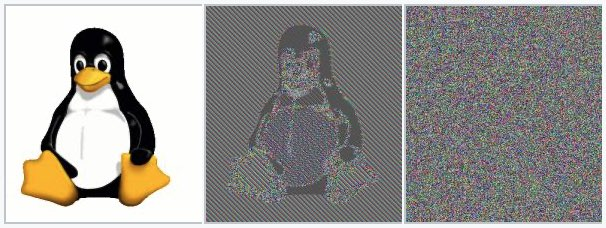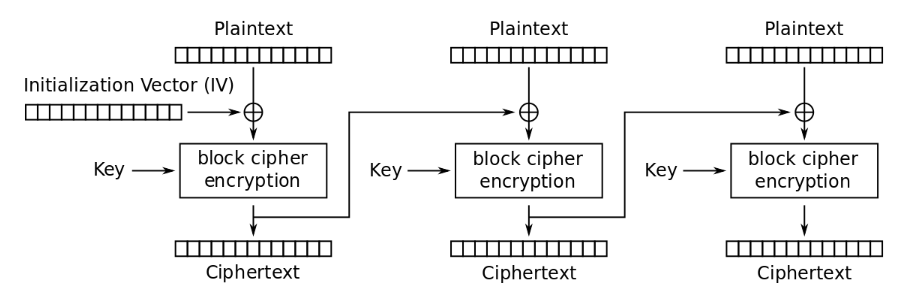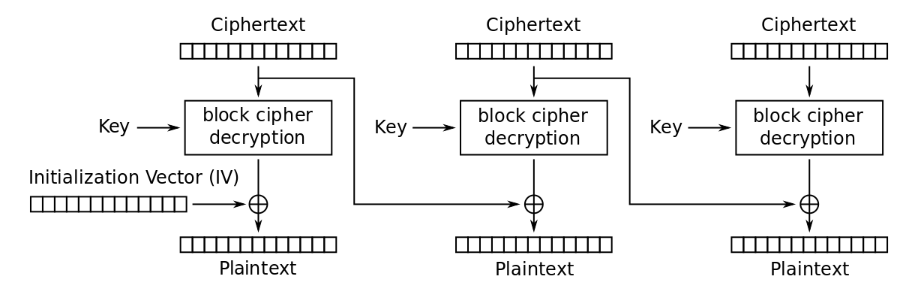Every secret creates a potential failure point.
Bruce Schneier（布鲁斯·施奈尔，美国密码学和信息安全专家、作家）

### 密文填充攻击简史

2002年，瑞士洛桑联邦理工学院的密码学家塞尔日·瓦德奈（Serge Vaudenay）在欧洲密码学年会（EUROCRYPT）上发表论文3，详述他发明的使用密文填充验证应答消息攻击CBC模式的对称分组加密密码。由于这种密文填充攻击的原理和实现都比较简单，同时CBC模式的分组密码应用非常普遍，瓦德奈的发明很快成为密码学家和信息安全专家的研究热点，也被许多黑客/极客投入实践中。在接下来的十多年里，源于这种攻击方法所发现的CBC模式相关的保密通信安全漏洞不断地涌现，如下所列：

• 2003年，结合时序攻击，破解IMAP电邮服务器与Outlook客户端之间的SSL/TLS加密信道，盗取用户密码。
• 2004年，证明攻击适用于ISO CBC模式加密和填充标准，可以有效地破译出明文。
• 2009年，利用消息认证码（MAC）执行时间的微小差别区分填充验证结果，攻破OpenSSL和GnuTLS的DTLS实现，恢复全部明文。
• 2012年，攻击硬件辅助RSA或CBC加密设备如USB安全令牌、智能卡和电子ID卡等，破解导入的密码。
• 2014年，谷歌安全团队报告“贵宾犬漏洞”（POODLE）。攻击者可利用软件实现中为照顾不同版本互通性而牺牲安全性的机制， 采用中间人攻击方式将客户端连接降级到SSL 3.0，再进行密文填充攻击破解密文。几个月后，又出现了一个新变种，攻击TLS 1.0-1.2服务器协议实现中的一些漏洞。
• 2016年，Steam游戏平台被曝光其客户端连接存在严重安全漏洞，可让攻击者组合重放攻击与密文填充攻击破解用户密码和其它保密信息；同年，常见漏洞与披露报告CVE-2016-2107揭示了OpenSSL针对“幸运十三攻击”的补丁中引入了另一个CBC模式密文填充攻击漏洞。

1. 采用认证加密方法，如具有最高安全性的Encrypt-then-MAC（EtM）：首先对明文进行加密，然后根据得到的密文生成MAC，密文和它的MAC一起发送；对密文的随意改动将造成消息认证错误，会被马上抛弃。比如SSHv2支持的hmac-sha1-etm@openssh.com算法。或者直接应用带有关联数据的认证加密（Authenticated Encryption with Associated Data，AEAD），既能保护数据私密性，又可以同时认证密文和明文。
2. 弃用TLS中的所有CBC密码套件（Cipher Suite），阻断密文填充攻击的可能性。如内容分发网络（Content Delivery Network, CDN）服务提供商Cloudflare从2016年开始全面转向支持分组加密AES-GCM和流加密ChaCha20-Poly1305，两者都是基于AEAD的密码套件。
3. 升级到TLS 1.2TLS 1.3，禁用之前版本的SSH/TLS协议，同时禁止降级协商，强制安全性高于互通性。TLS 1.3不允许任何非AEAD加密算法。2021年3月，IETF发布当前最佳实践RFC 8996，正式宣布弃用 TLS 1.0 和 TLS 1.1。根据SSL Labs2021年9月的扫描统计，在全球最流行150,000个启用SSL/TLS的网站中99.6%支持TLS 1.2，48.9%的站点支持TLS 1.3。

### AES-CBC工作模式

#### AES标准

• 密钥长度128比特，10轮
• 密钥长度192比特，12轮
• 密钥长度256比特，14轮

#### 填充格式

    2.   Some content-encryption algorithms assume the
input length is a multiple of k octets, where k > 1, and
let the application define a method for handling inputs
whose lengths are not a multiple of k octets. For such
algorithms, the method shall be to pad the input at the
trailing end with k - (l mod k) octets all having value k -
(l mod k), where l is the length of the input. In other
words, the input is padded at the trailing end with one of
the following strings:
01 -- if l mod k = k-1
02 02 -- if l mod k = k-2
.
.
.
k k ... k k -- if l mod k = 0

0 0x10 10 10 10 10 10 10 10 10 10 10 10 10 10 10 10 10*
1 0x01 XX XX XX XX XX XX XX XX XX XX XX XX XX XX XX 01
2 0x02 XX XX XX XX XX XX XX XX XX XX XX XX XX XX 02 02
... ... ....
14 0x0E XX XX 0E 0E 0E 0E 0E 0E 0E 0E 0E 0E 0E 0E 0E 0E
15 0x0F XX 0F 0F 0F 0F 0F 0F 0F 0F 0F 0F 0F 0F 0F 0F 0F

TLS协议为CBC分组密码套件所指定的填充格式与PKCS #7大同小异。TLS 1.2记录层协议规范定义了如下类C语言的区块结构：

TLS协议规定用户数据、MAC、填充序列及填充长度一起构成待加密的明文block-ciphered。这里填充长度是PKCS #7所没有的，总是位于最后一个字节。填充序列字节数值就是填充长度。相应地，TLS协议下AES-CBC的填充格式变为：

0 XX XX XX XX XX XX XX XX XX XX XX XX XX XX XX 00
1 0x01 XX XX XX XX XX XX XX XX XX XX XX XX XX XX 01 01
2 0x02 XX XX XX XX XX XX XX XX XX XX XX XX XX 02 02 02
... ... ...
14 0x0E XX 0E 0E 0E 0E 0E 0E 0E 0E 0E 0E 0E 0E 0E 0E 0E
15 0x0F 0F 0F 0F 0F 0F 0F 0F 0F 0F 0F 0F 0F 0F 0F 0F 0F*

#### 工作模式（来源：维基百科条目“分组密码工作模式”）CBC加密流程（来源：维基百科条目“分组密码工作模式”）CBC解密流程（来源：维基百科条目“分组密码工作模式”）

CBC工作模式的加密过程可以用数学公式表示为：

\begin{align} C_0&=IV\\ C_i&=E_K(P_i⊕C_{i-1})\tag{1} \end{align}

\begin{align} C_0&=IV\\ P_i&=D_K(C_i)⊕C_{i-1}\tag{2} \end{align}

### 理解密文填充攻击

#### 攻击破解密文

1. 确定尾块填充的长度：对于最后一个区块（即尾块）$$C_n$$，可以肯定的是它一定包含填充，最少一个0x01，最多16个0x10（参见前面表1）。攻击者从$$C_{n-1}$$的左边第一个字节开始，将其修改成 $C_{n-1}'=C_{n-1}⊕{\mathrm {0x10}}\tag{4}$ 然后将$$C_{n-1}'$$$$C_n$$一起发给接收方。接收方执行AES-CBC解密流程得到左边第一个字节为 \begin{align} P_n^*&=P_n'⊕C_{n-1}'\\ &=P_n'⊕(C_{n-1}⊕{\mathrm {0x10}})\\ &=(P_n'⊕C_{n-1})⊕{\mathrm {0x10}}\\ &=P_n⊕{\mathrm {0x10}} \end{align} 以上推演基于异或运算满足交换律的特性。这时如果接收方报告“填充无效”，表明$$P_n^*$$为填充的一部分，填充长度一定是16。如果接收方没有返回此错误，那么$$C_{n-1}$$的变化不影响填充验证，填充长度一定小于16，攻击者再按照$$(4)$$式修改第二个字节，再次发给接收方。这时如果接收方报告“填充无效”，则填充长度一定是15。依此类推，从左到右逐字节递进，攻击者就可以得出尾块填充的确切长度。

2. 破解尾块数据明文：得到尾块填充长度后，攻击者就可以从右到左逐个破解尾块数据明文。假设填充长度为$$L$$，由PKCS #7规范得出明文块最后$$L$$个字节数值全为$$L$$，为通用起见记为$$P_n[j]=M$$。攻击者先如下修改$$C_{n-1}$$的最后$$L$$个字节 $C_{n-1}'[j]=C_{n-1}[j]⊕M⊕(L+1)\qquad j=(16-L),\cdots,15\tag{5}$ 如此接收方执行AES-CBC解密后得到最后$$L$$个字节为 \begin{align} P_n^*[j]&=(P_n'[j]⊕C_{n-1}[j])⊕M⊕(L+1)\\ &=P_n[j]⊕M⊕(L+1)\\ &=M⊕M⊕(L+1)\\ &=L+1 \end{align} 所以这样做的结果等于强制解密后最后$$L$$个字节为$$L+1$$。然后，攻击者尝试对$$C_{n-1}$$倒数第$$L+1$$个字节（就是下一个要破解的字节）执行 \begin{align} C_{n-1}'[15-L]&=C_{n-1}[15-L]⊕X\tag{6}\\ P_n^*[15-L]&=(P_n'[15-L]⊕C_{n-1}[15-L])⊕X\\ &=P_n[15-L]⊕X\tag{7}\\ \end{align} $$X$$的取值范围为0x00-0xFF。在这个区间有且仅有一个$$X$$，使得$$P_n^*[15-L]$$$$L+1$$，即倒数第$$L+1$$个字节也为$$L+1$$。这时整个区块以$$L+1$$$$L+1$$结尾，是唯一攻击者不会收到“填充无效”应答的情况。根据$$(7)$$式导出 $P_n[15-L]=(L+1)⊕X\tag{8}$ 所以只要找到$$X$$，攻击者马上可以算出明文倒数第$$L+1$$个字节为$$(L+1)⊕X$$。接下来将$$L$$递增，重复此过程，继续破解倒数第$$L+1$$个明文字节。如下，攻击者就能破解尾块全部数据字节：

可以看出，在这种攻击方法下破解每一个明文字节XX平均需要发送128次修改的密文。这是很有效的。

3. 破解非尾块数据明文：对于非尾部区块，即$$i<n$$时，攻击的方式没有什么本质不同。攻击者依照$$(6)$$式更改$$C_{i-1}$$的最后一个字节（即$$C_{i-1}'$$），然后发送$$(C_{i-1}',C_i)$$至服务器。这时$$C_i$$会被视为尾块，当接收方返回“填充无效”的消息，攻击者尝试下一个$$X$$。当接收方没有返回“填充无效”的消息时，需要区分下面几种情况：

• 如果攻击者事先知道明文数据字节都大于0x10，比如都是ASCII表中可打印的字符，那么可以确信解密后的最后一个字节$$P_i^*$$为0x01。这是因为系统认定填充一定存在，而0x01是唯一可能通过验证的单字节填充情况。根据$$(8)$$式得到${P_i=\mathrm {0x01}}⊕X\tag{9}$就此破解了最右边字节。

• 如果明文数据字节没有取值范围限制，则解密后的最后一个字节$$P_i^*$$要么为0x01，要么为0x02-0x10之间的某个值。前者一定会出现，后者会碰巧当该明文块数据为以下15种之一的式样时发生

这时接收方一样也认为填充正确。如何分辨这两者呢？有一个简单的办法。攻击者在没有收到“填充无效”消息时，修改$$C_{i-1}$$的倒数第二个字节$$C_{i-1}$$，再重发给接收者。如果是前者，接收方还是不会返回“填充无效”的消息，因为倒数第二个字节的变化对此没有影响；如果是后者，倒数第二个字节的变化破坏了填充格式，接收方返回“填充无效”的消息。攻击者鉴别出前者后，应用$$(9)$$式就可以了。

接下来对非尾块其它数据明文字节的破解，遵照上面第二步（破解尾块数据明文）相同的处理，参考$$(5-8)$$式从右到左逐个字节破解。说明一下，对第一个密文块$$C_1$$，攻击需要的前一块$$C_0$$就是$$IV$$。如果攻击者无法获取$$IV$$，就不能破解$$C_1$$

总结统计攻击非尾块时，如果明文数据字节都大于0x10，破解一个AES-CBC密文块平均需要发送2048（128x16）次查询；如果明文数据字节没有取值范围限制，则额外需要多一次查询。

#### CBC-R伪造明文

CBC密文填充攻击的目的是破解截获的密文，从而无需密钥就可以恢复明文。那么它可以用来伪造能被对方接受的明文吗？两位信息安全专家Juliano Rizzo和Thai Duong给出了肯定的答案4。仔细观察$$(3)$$式，很显然密文填充攻击完全恢复了明文$$P_i$$和中间结果$$P_i'$$。作为选择密文攻击，攻击者当然可以任意更改$$C_{i-1}$$以控制接收方解密后所见的$$P_i$$。也就是说，攻击者可以利用CBC密文填充攻击，在密钥未知的情况加密任意长度的消息。

Rizzo和Duong为这种变种攻击设计了流程，并命名为CBC-R（R代表Reverse，即逆向操作）。CBC-R的流程如下图所示1. 选择要构造的明文消息，分割成$$N$$个16字节块$$P_1,P_2,..,P_n$$
2. 选择随机字节$$r_1,r_2,..,r_{16}$$，设定$$C_n=r_1|r_2|...|r_{16}$$
3. $$i=n$$ 递减到 $$2$$:
$$C_{i−1}=P_i⊕D_{PaddingOracle}(C_i)$$
4. $$IV=P_1⊕D_{PaddingOracle}(C_1)$$
5. 输出$$IV$$和密文序列$$C =C_1|C_2|...|C_n$$

1. 无效填充
2. 填充正确，HMAC错误
3. 填充正确，HMAC正确

### Python编程实现

#### 填充及验证函数

PKCS #7填充规则的Python实现很直观，两行程序就可以了。第一行将数据字节长度对区块长度取余，再将余数从区块长度中减去，得到的就是填充字节的长度，也是填充字节本身的数值；第二行函数返回尾部添加重复填充后的字节序列：

PKCS #7填充的验证和去除也很简单。我们可以自定义特殊类型的填充异常类，然后根据不同的出错条件引发。出错条件包括：

1. 数据字节总长度不是区块长度的整数倍
2. 填充字节本身的数值为0或大于区块长度
3. 数据字节序列尾部并非重复填充后的字节

#### 加密和解密函数

AES-128加解密函数就绪之后，参考CBC工作模式下加解密的流程图，就可以应用迭代很快写出AES-CBC-128的加解密函数了：

#### 攻击程序汇总

1. 调用get_random_string()生成随机长度可打印字符串
2. 将该字符串转化为明文字节序列
3. 调用库函数urandom()生成随机IV和密钥
4. 调用aes_128_cbc_encrypt()加密明文字节序列
5. 调用split_bytes_blocks()分割上一步输出的密文字节序列，输出密文块列表
6. 将IV转化为字节序列，添加到上一步生成的密文块列表的头部
7. 将密文块列表的最后两个块输入到crack_padding_length()，得出尾块填充长度
8. 启动循环，从密文块列表尾部开始，调用crack_cipher_block()逐个破解
9. 调用pkcs7_stripping()从破解的明文块尾部去除PKCS #7填充
10. 比较破解出的明文和原始明文字节序列

1. D. Bleichenbacher. Chosen Ciphertext Attacks Against Protocols Based on the RSA Encryption Standard PKCS#1. In Advances in Cryptology CRYPTO’98, Santa Barbara, California, U.S.A., Lectures Notes in Computer Science 1462, pp. 1–12, Springer-Verlag, 1998.↩︎

2. J. Manger. A Chosen Ciphertext Attack on RSA Optimal Asymmetric Encryption Padding (OAEP) as Standardized in PKCS#1 v2.0. In Advances in Cryptology CRYPTO’01, Santa Barbara, California, U.S.A., Lectures Notes in Computer Sci- ence 2139, pp. 230–238, Springer-Verlag, 2001.↩︎

3. S. Vaudenay, Security Flaws Induced by CBC Padding - Applications to SSL, IPSEC, WTLS.... In Advances in Cryptology- EUROCRYPT 2002. Springer, 2002, pp. 534–545.↩︎

4. J. Rizzo; T. Duong, Practical Padding Oracle Attacks. USENIX WOOT 2010.↩︎